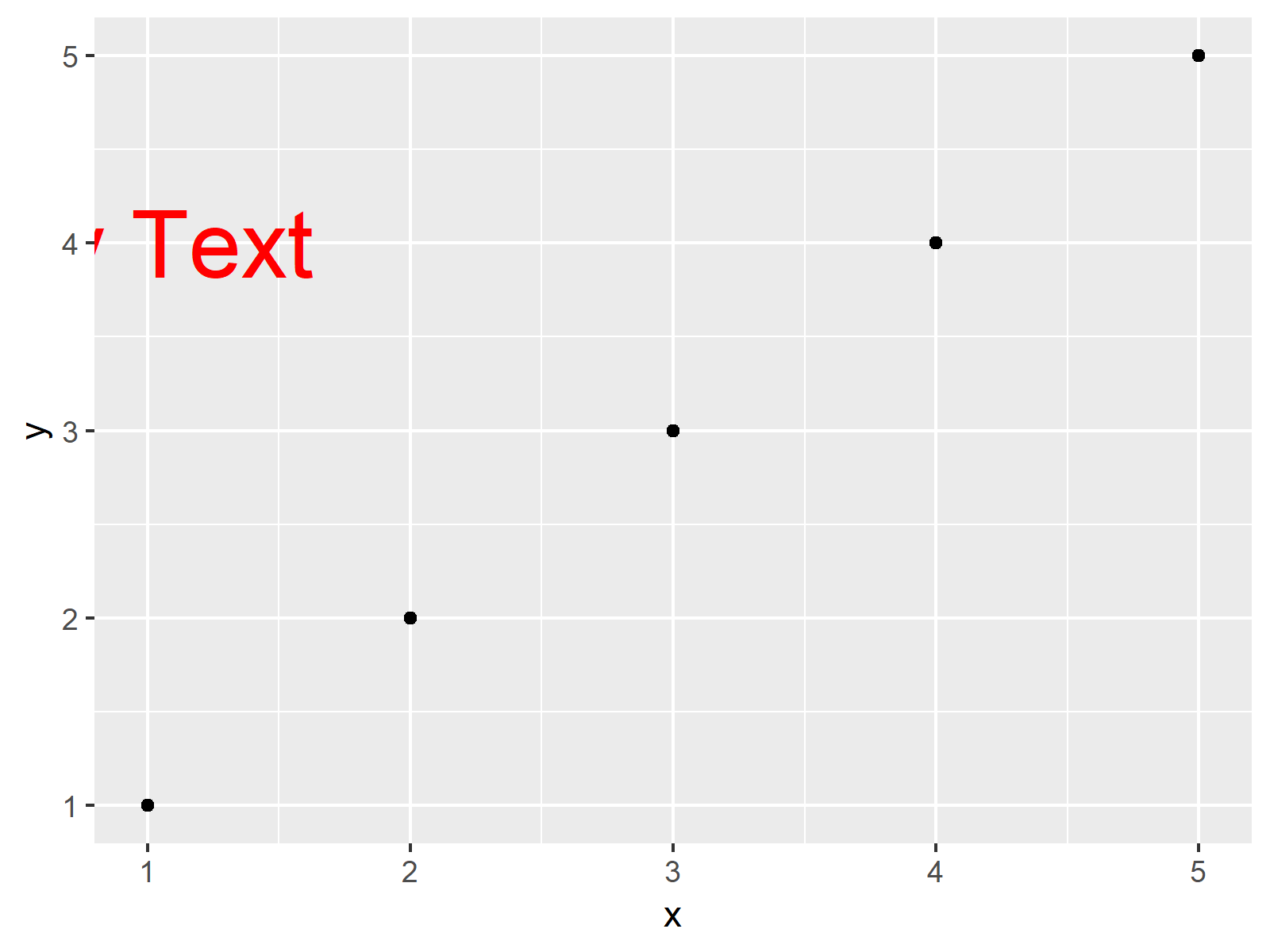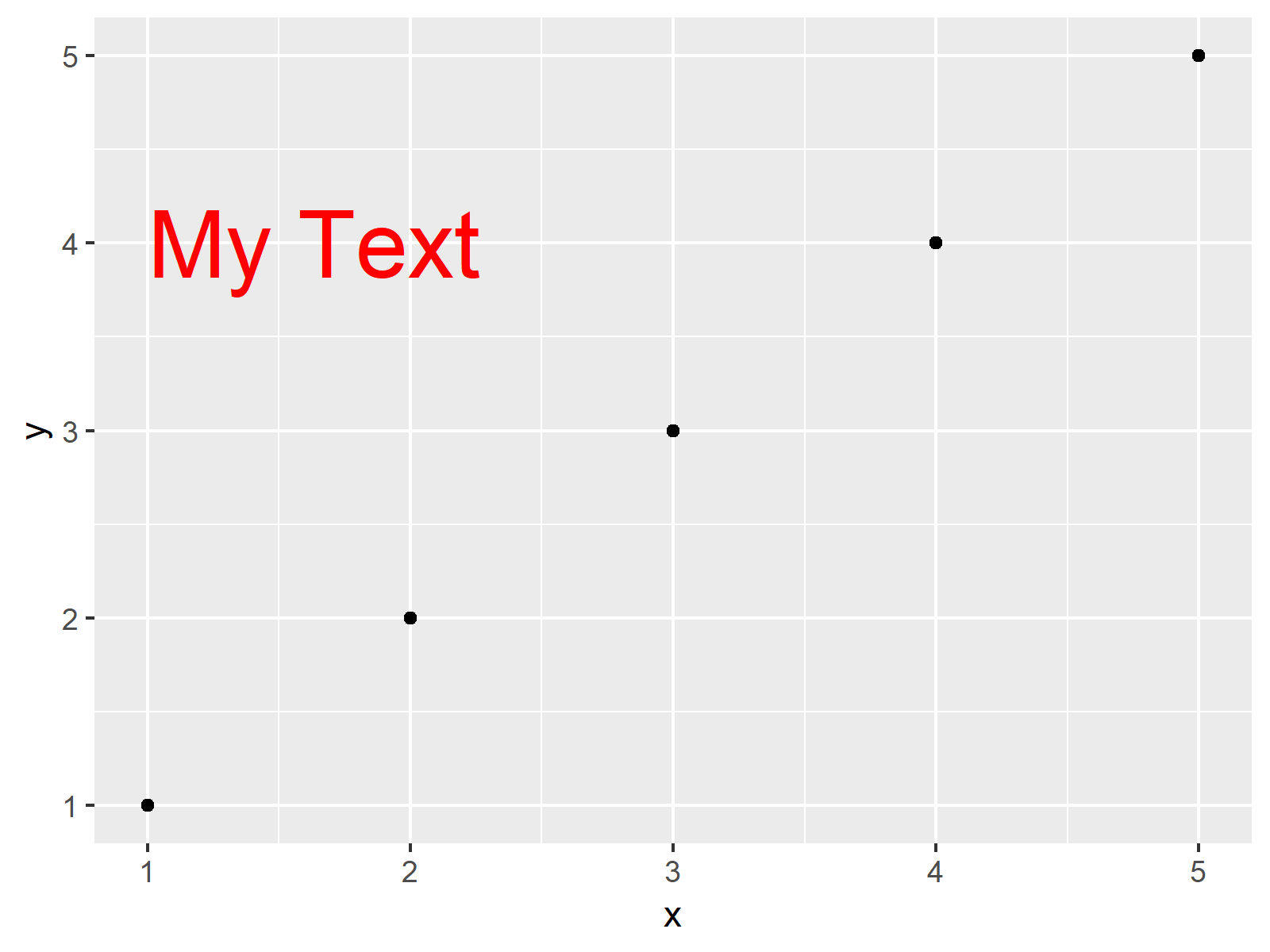# Left-Align Text in ggplot2 Plot in R (Example)

In this article, you’ll learn how to align text in a ggplot2 graphic on the left side of the plot in R.

It’s time to dive into the example:

## Example Data, Packages & Basic Plot

Consider the following example data.

```data <- data.frame(x = 1:5,         # Example data
y = 1:5)
data                                # Print data
#   x y
# 1 1 1
# 2 2 2
# 3 3 3
# 4 4 4
# 5 5 5```

As you can see based on the previously shown output of the RStudio console, our example data consists of two numeric columns and five rows.

If we want to use the functions of the ggplot2 package, we also have to install and load ggplot2:

```install.packages("ggplot2")         # Install & load ggplot2 package
library("ggplot2")```

Now, we can draw our data as follows.

```ggp <- ggplot(data, aes(x, y)) +    # Create basic ggplot2 plot
geom_point()
ggp                                 # Print plot```The output of the previously shown R code is shown in Figure 1: It illustrates a basic ggplot2 scatterplot in R. We have not added any text elements yet.

## Example: Add Left-Aligned Text to ggplot2 Plot

In this Example, I’ll illustrate how to draw left-aligned text to a ggplot2 plot in R.

```ggp +                               # Add text to plot (text is cut-off)
annotate(geom = "text",
x = 1, y = 4,
label = "My Text",
col = "red",
size = 10)```The output of the previously shown R programming code is shown in Figure 2. As you can see, we have added some text to the plot using the annotate function. However, you can also see that this text is cut-off on the left side. Let’s change this!

The R code below is just modified slightly by adding the hjust argument within the annotate function. If we set the hjust argument to be equal to zero, our text is getting aligned on the left side of the plot.

```ggp +                               # Add text to plot (text is NOT cut-off)
annotate(geom = "text",
x = 1, y = 4,
label = "My Text",
col = "red",
size = 10,
hjust = 0)               # Adding hjust argument```As shown in Figure 3, the previous R code plotted a ggplot2 graph with left-aligned text. Looks good!

## Video & Further Resources

Have a look at the following video which I have published on my YouTube channel. In this tutorial, I give a detailed introduction to the ggplot2 Package and data visualization in R, structured in different sections with examples for beginners but also advanced users.

Please accept YouTube cookies to play this video. By accepting you will be accessing content from YouTube, a service provided by an external third party.If you accept this notice, your choice will be saved and the page will refresh.

Furthermore, you may want to have a look at some of the other tutorials that I have published on this website.

Summary: This page explained how to left-align a ggplot2 plot using the annotate function and the hjust argument in R programming. Don’t hesitate to let me know in the comments section below, in case you have further questions. Besides that, please subscribe to my email newsletter for updates on new tutorials.

Subscribe to the Statistics Globe Newsletter